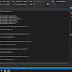Calculator in c# console Is a project on the concept of normal mathematical operation on the calculator. c# calculator source code contains the number, operators, and sign like in the normal calculator. in this project, the user has to select the operation at first that they want in the calculation.

## Calculator in C# Console Application

Calculator in a console application is a simple C# programming language. C# application is calculating enters number to any operators and also capable of handling all type calculations. Here the user has to add all the operators on day one in the calculator then they can use calculation.

### How To Run The Project?

The order to run this project you have must install visual studio 2019 on your PC. and you can console the calculator in this project and open source code your Visual Studio then run your project.Share it: